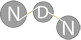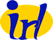NS-3 based Named Data Networking (NDN) simulator ndnSIM 2.5: NDN, CCN, CCNx, content centric networks API Documentation
ns3::GaussMarkovMobilityModel Class Reference

`#include <introspected-doxygen.hpp>`

## Detailed Description

### Config Paths

ns3::GaussMarkovMobilityModel is accessible through the following paths with Config::Set and Config::Connect:

• "/NodeList/[i]/\$ns3::MobilityModel/\$ns3::GaussMarkovMobilityModel"

### Attributes

• Bounds: Bounds of the area to cruise.
• Set with class: BoxValue
• Underlying type: Box
• Initial value: -100|100|-100|100|0|100
• TimeStep: Change current direction and speed after moving for this time.
• Set with class: ns3::TimeValue
• Underlying type: Time -9.22337e+18ns:+9.22337e+18ns
• Initial value: +1e+09ns
• Alpha: A constant representing the tunable parameter in the Gauss-Markov model.
• Set with class: ns3::DoubleValue
• Underlying type: double -1.79769e+308:1.79769e+308
• Initial value: 1
• MeanVelocity: A random variable used to assign the average velocity.
• MeanDirection: A random variable used to assign the average direction.
• MeanPitch: A random variable used to assign the average pitch.
• NormalVelocity: A gaussian random variable used to calculate the next velocity value.
• NormalDirection: A gaussian random variable used to calculate the next direction value.
• NormalPitch: A gaussian random variable used to calculate the next pitch value.

### Attributes defined in parent class ns3::MobilityModel

• Position: The current position of the mobility model.
• Set with class: Vector3DValue
• Underlying type: Vector3D
• Velocity: The current velocity of the mobility model.
• Set with class: Vector3DValue
• Underlying type: Vector3D